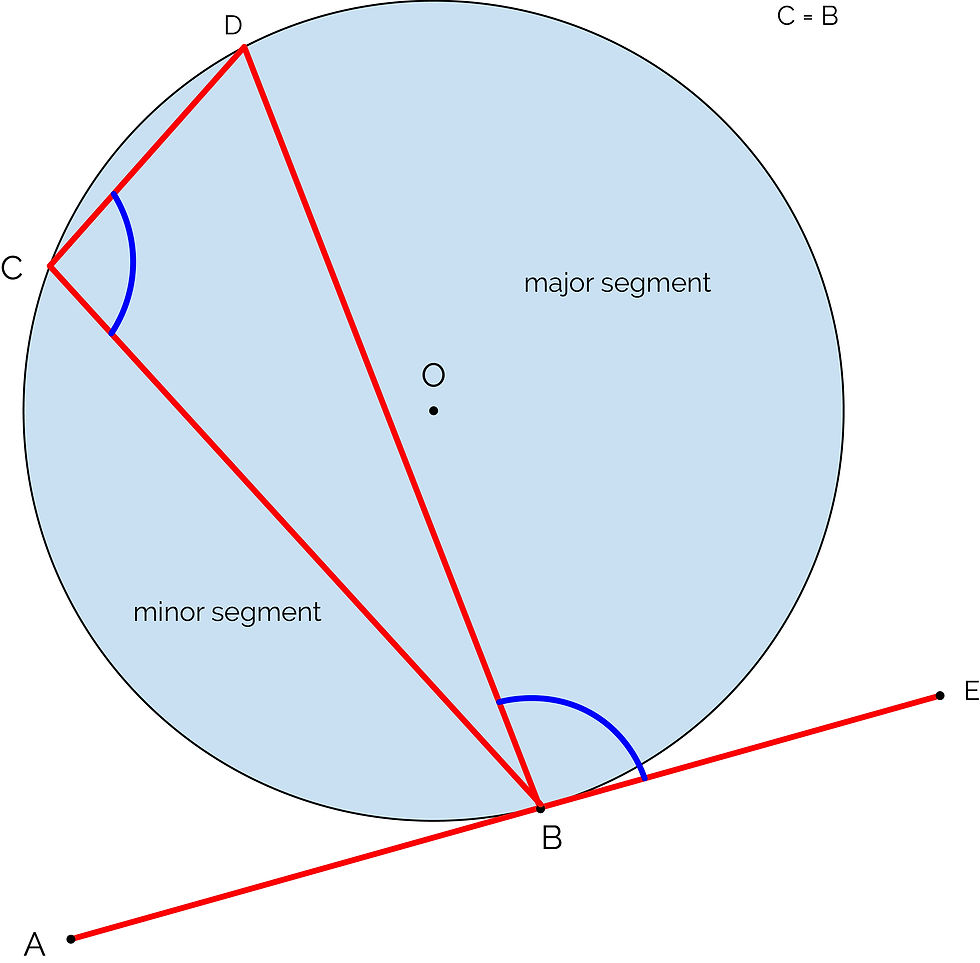cum
top of page
Search

# CSEC Mathematics: Circle Theorem

You should already be familiar with the important parts of a circle:Circle theorem helps us to find and prove the sizes of angles in relation to circles. You may be wondering, what is the point of circle theorem? How is it useful in everyday life? Will it benefit me in my career?

Well, I can't tell you that its the most useful concept in the world, but, I also can't tell you how many times it's been useful for me to know an angle in a circle when I'm popping some cold ones with the boys.

Theorem 1The angle subtended by an arc at the center of the circle is twice the size of the angle subtended at the circumference.

The term 'subtend' basically means to form an angle by drawing lines from the two end points. So, the arc (AC) subtends both angle D and B.

Theorem 2The angle subtended by the diameter is 90°. This is really a special case of theorem 1, as the angle at the center would be 180°. So, the angle at the circumference is 90°.

Theorem 3Angles at the circumference in the same segment of a circle and subtended by the same arc/chord are equal. Both angles are in the major segment and are subtended by the same arc/chord (AE)

Theorem 4The opposite angles in a cyclic quadrilateral are supplemetary (add up to 180°). A cyclic quadrilateral is a quadrilateral that has all of its vertices on the circumference of the circle.

Theorem 5The lengths of two tangents from an external point to the points of contact on the circle are equal.

AB = CB

Theorem 6The angle between a tangent and a radius in a circle is 90°.

Theorem 7The angle between a tangent to a circle and a chord through the point of contact is equal to the angle in the alternate segment.

You can see how this works for yourself here:

Theorem 8The line joining the centre of a circle to the midpoint of a chord is perpendicular to the chord. This can also be worded as saying that the radius bisecting a chord is perpendicular to the chord, or a line from the center of a circle perpendicular to the chord divides the chord into two equal parts.